# Rajasthan Board Question Paper for Class 10 Maths 2017 In PDF

## RBSE 10th Maths Question Papers 2017 With Solutions

Rajasthan Board 10th Maths 2017 question paper with solutions in PDF and text format for Maths in English Medium will help the students for their board exams. They can also download the pdf version of maths question paper 2017 Class 10 for reference. Along with this, Students can download the past year’s RBSE Class 10 Question Papers for Maths from Rajasthan board previous year maths question papers.

These papers will help the candidates to score good marks. RBSE 10th Maths 2017 question paper can be downloaded without any efforts, and students can practice by verifying the answers provided by BYJU’S. It is necessary for the students to understand the new pattern and style of question paper according to the latest exam pattern. We hope RBSE 10th Maths 2017 question paper will be of greater help to prepare for the upcoming RBSE Board Exams.

### QUESTION PAPER CODE S–09–Mathematics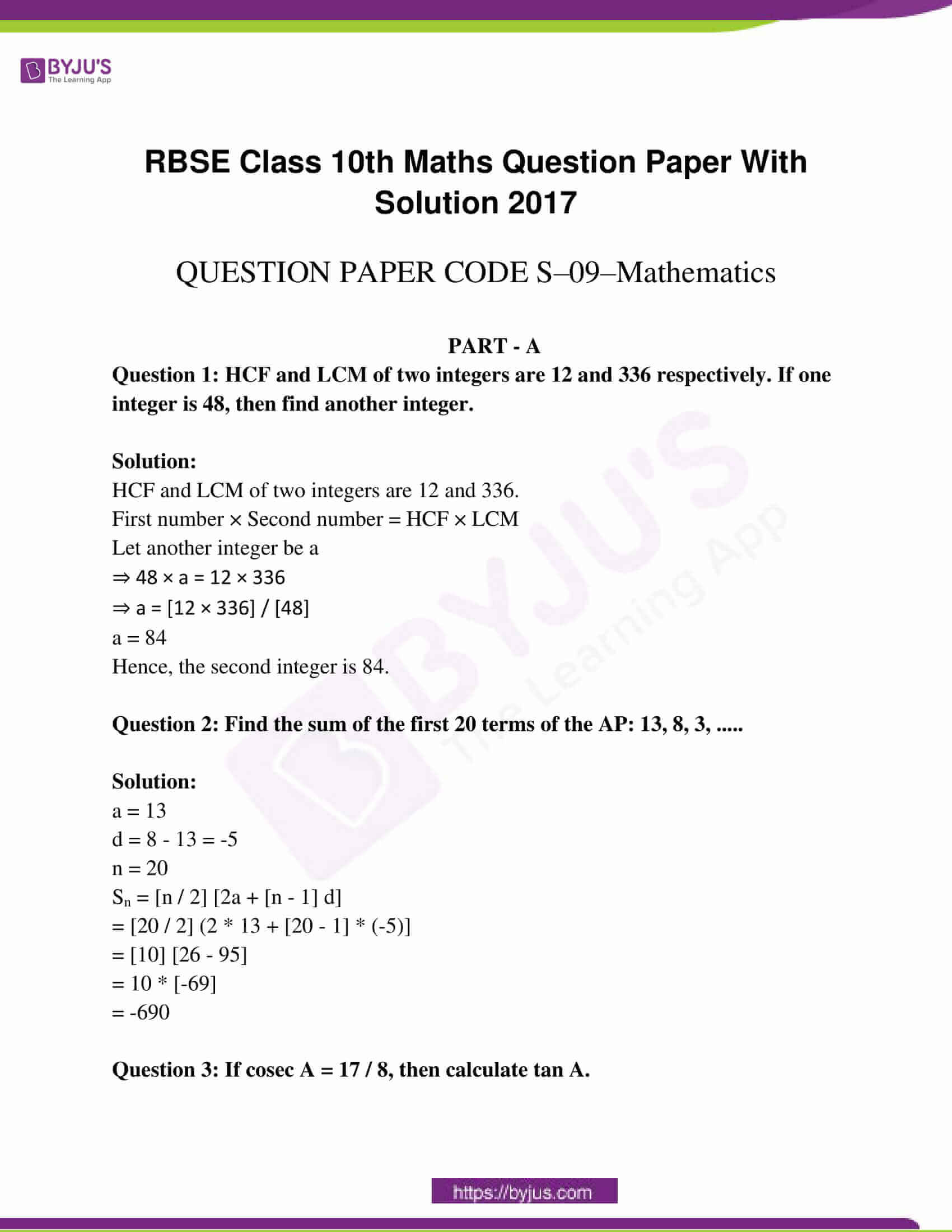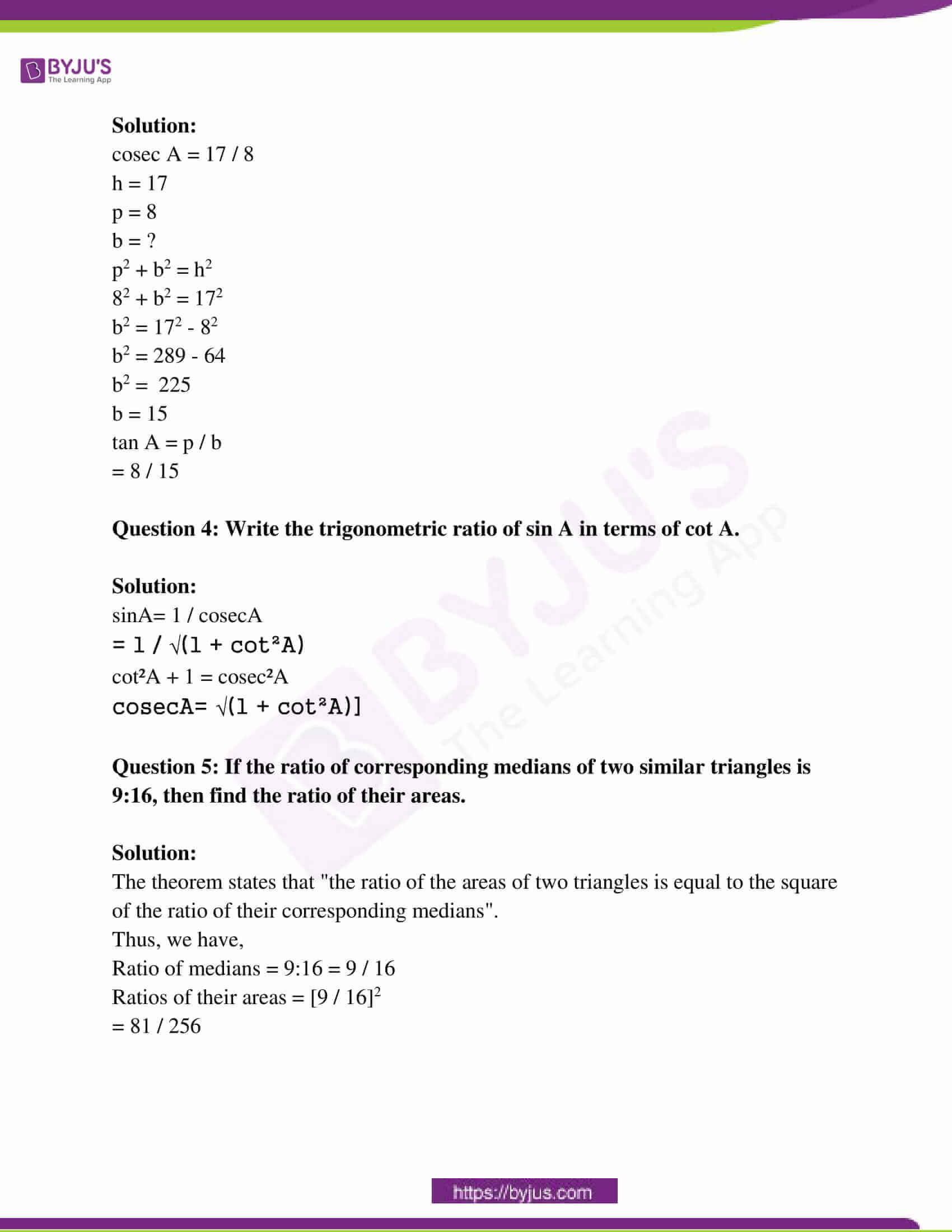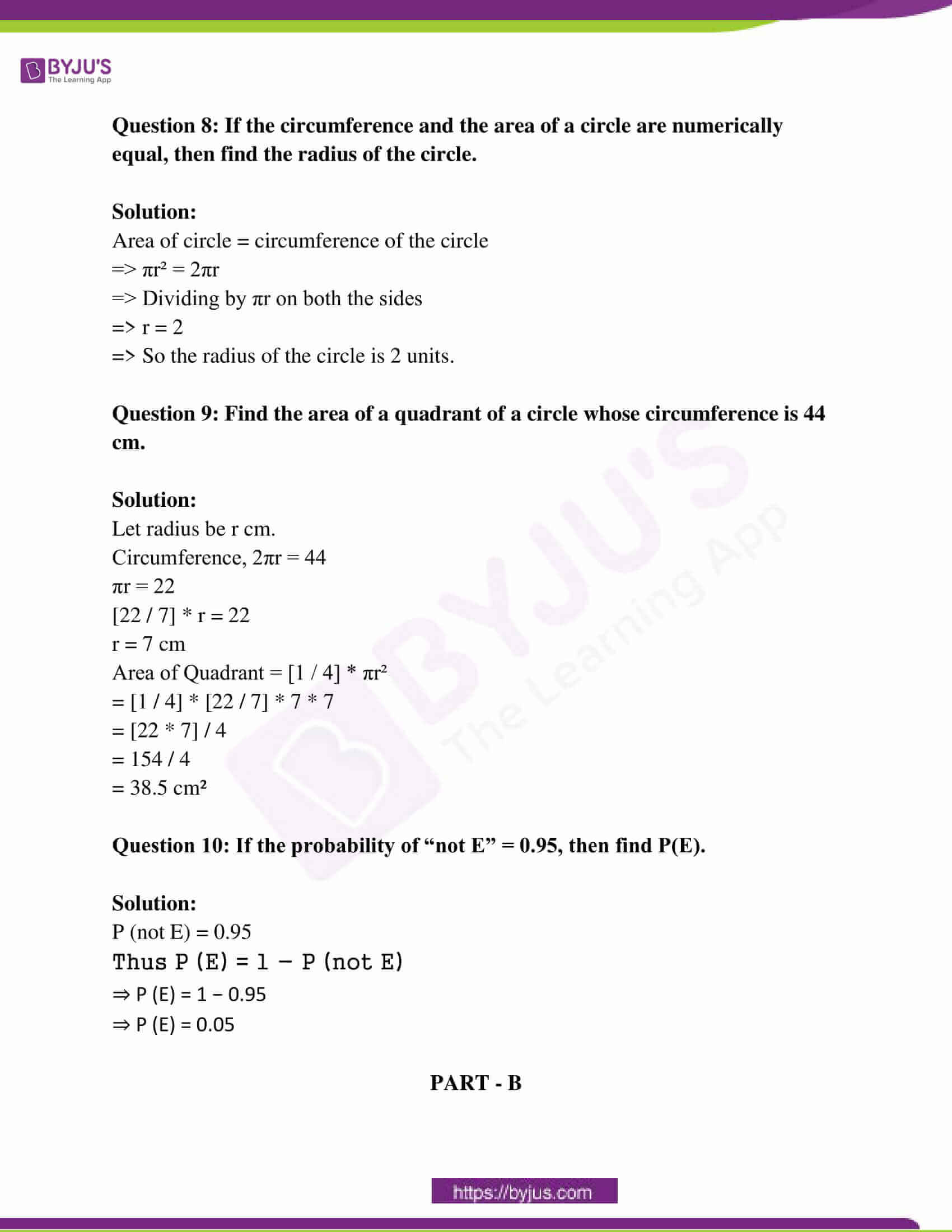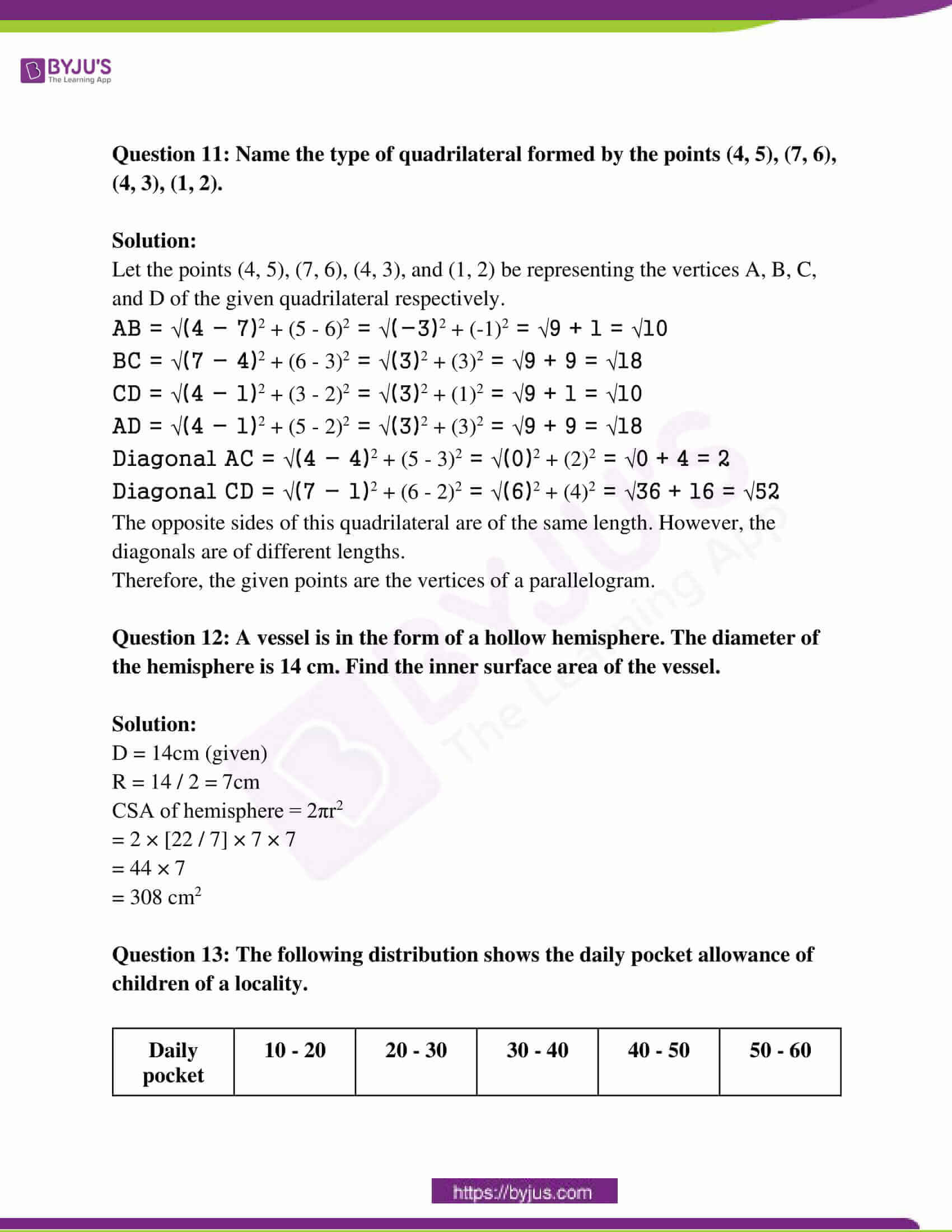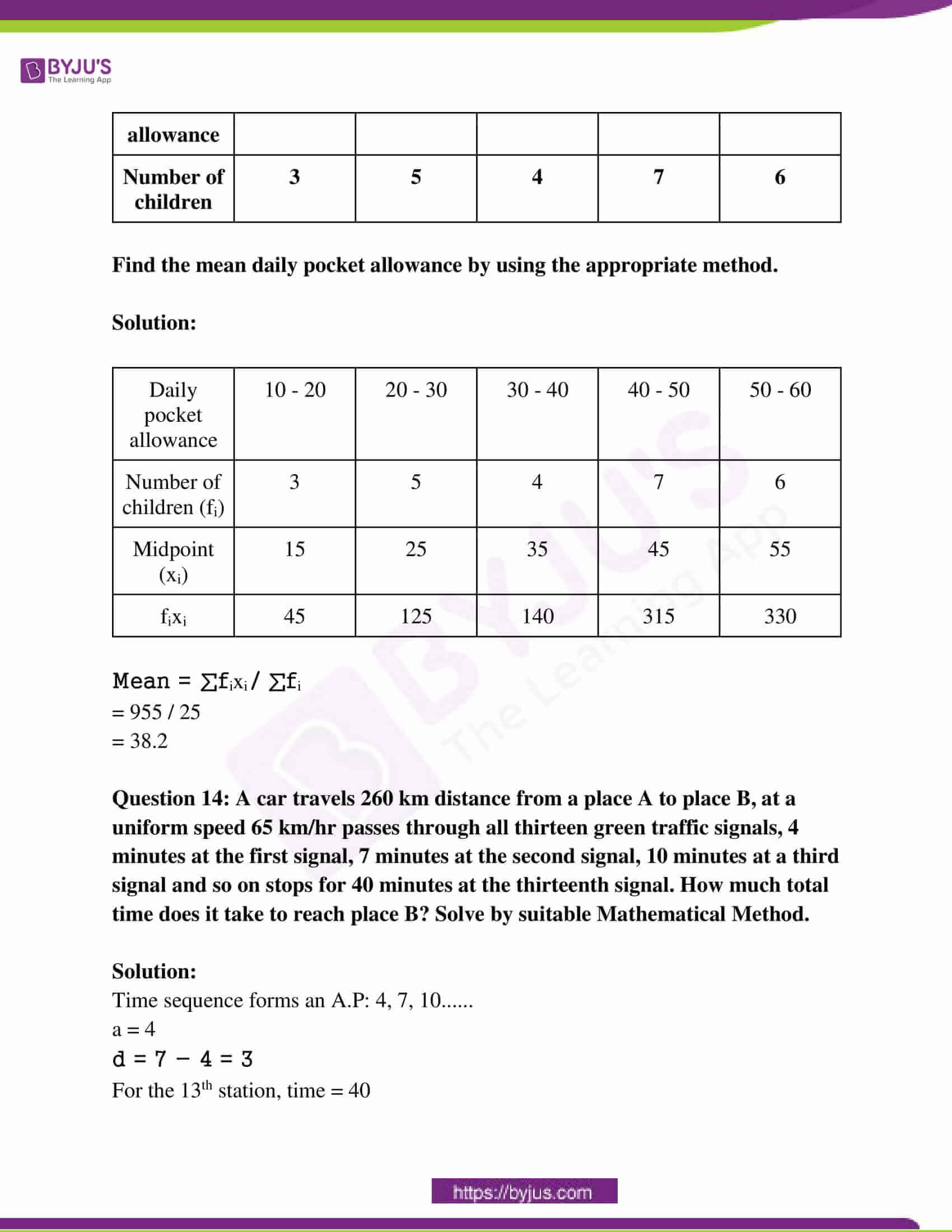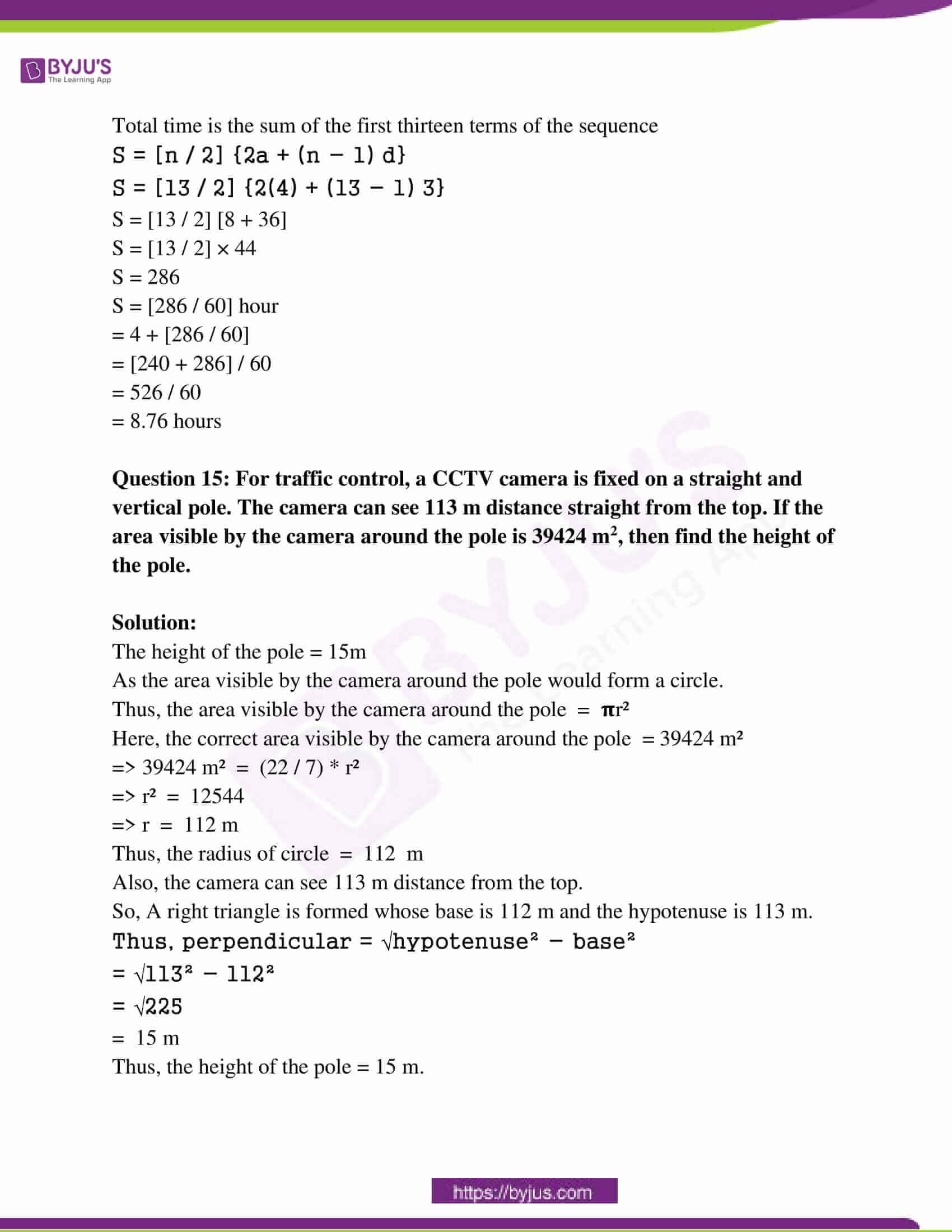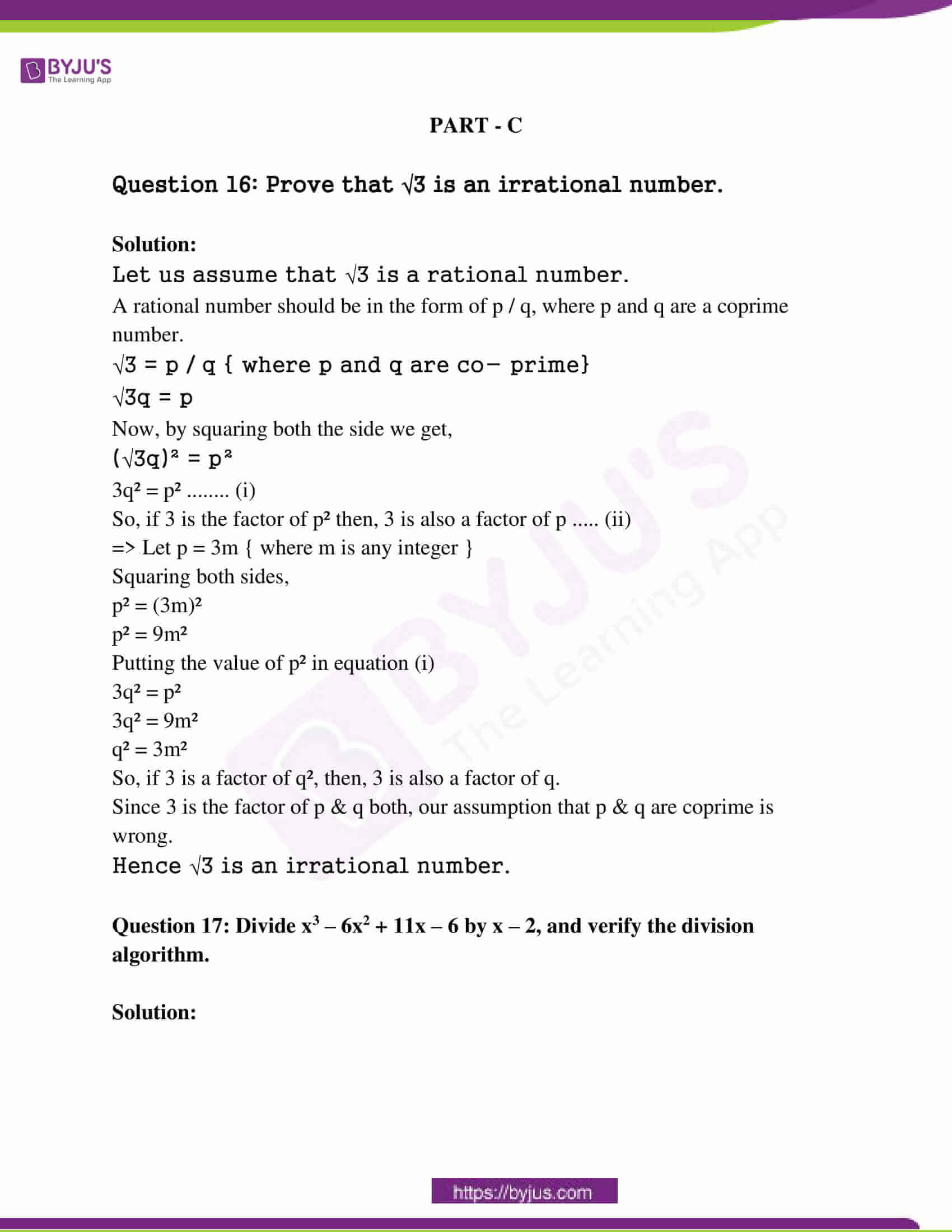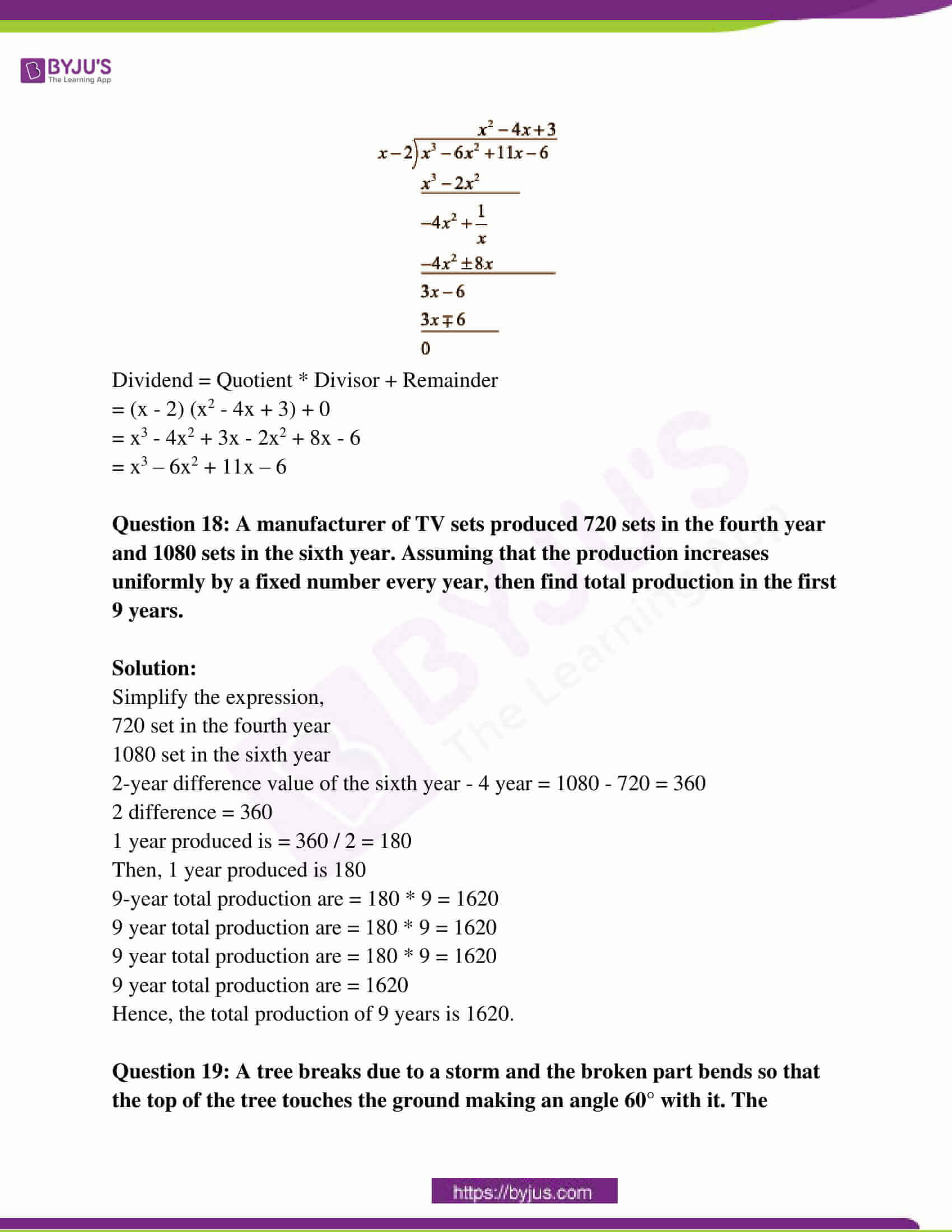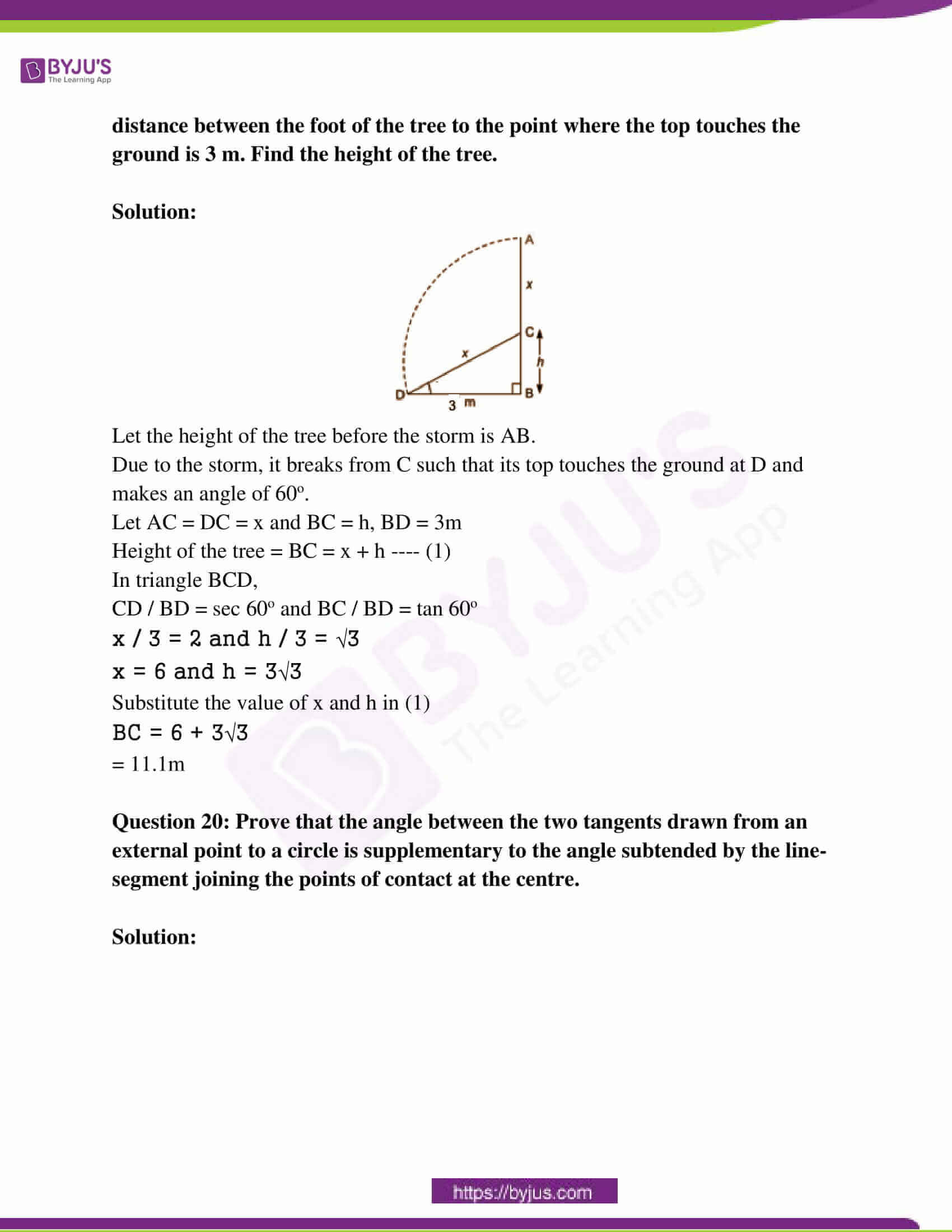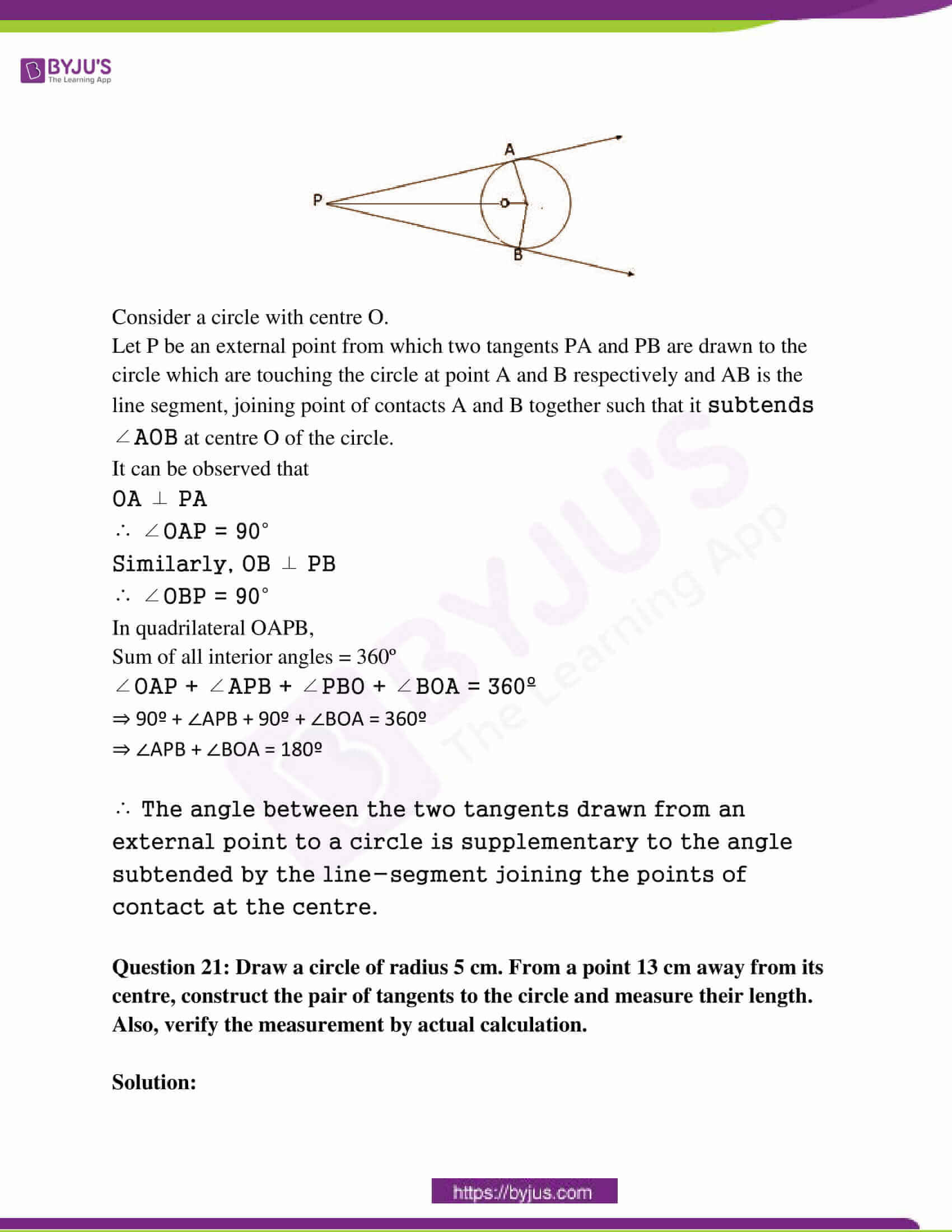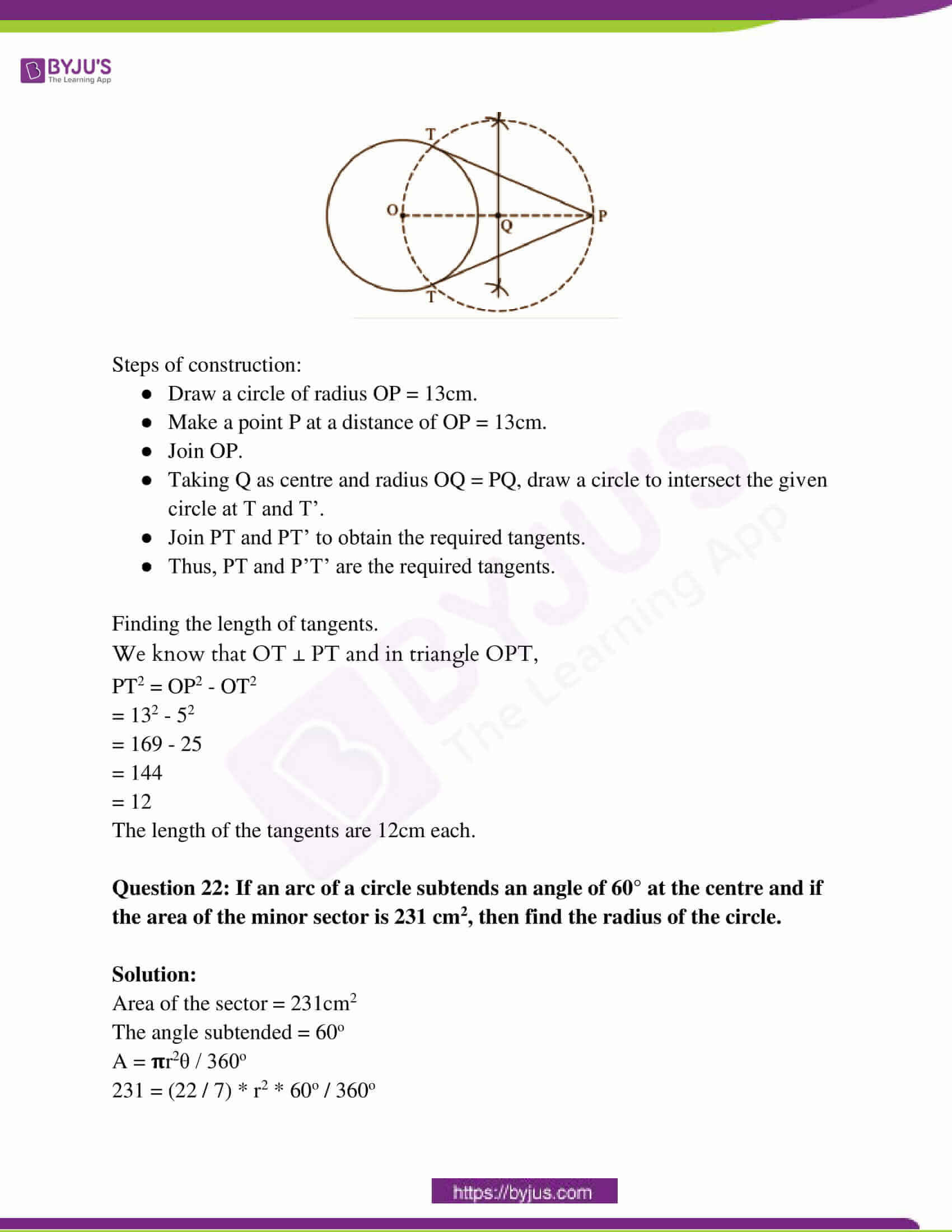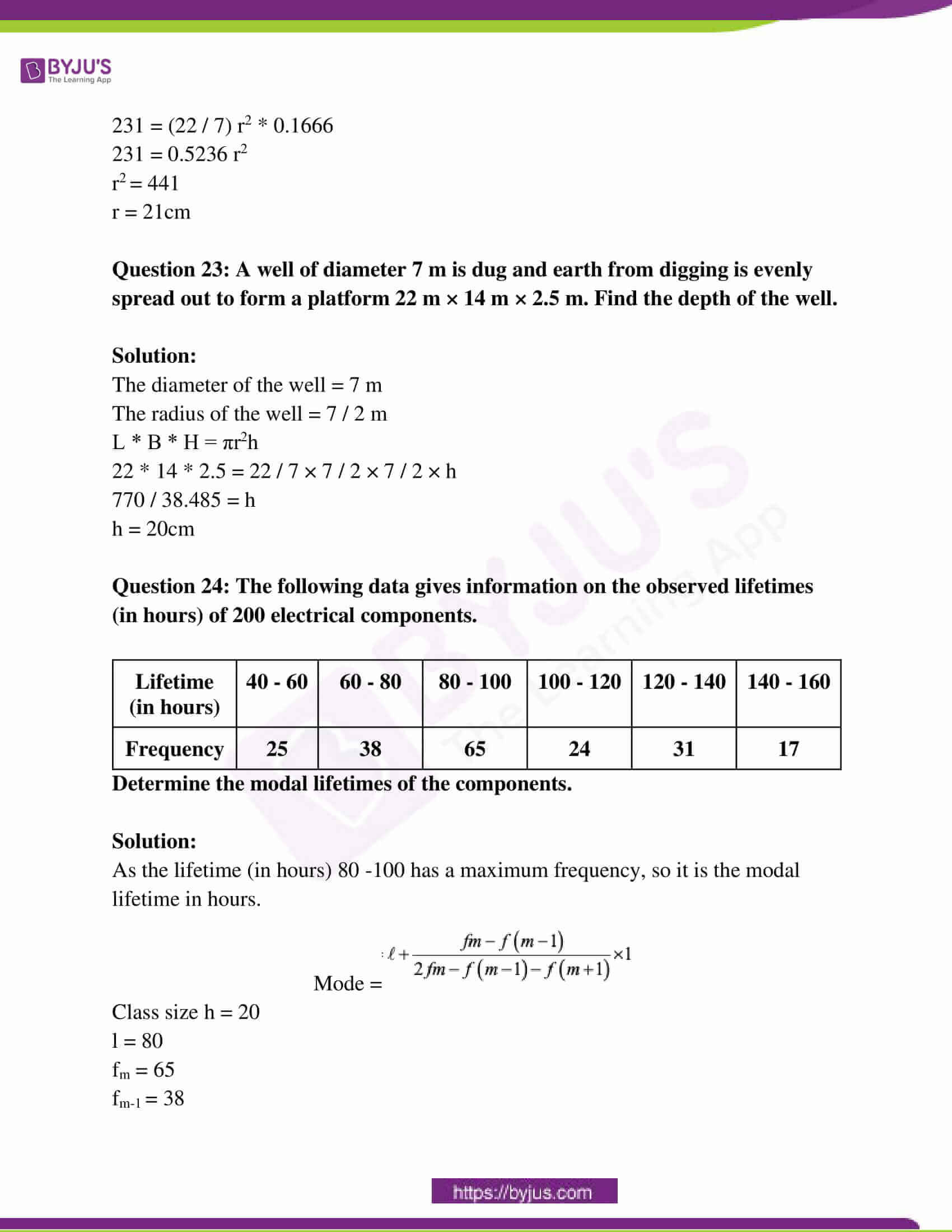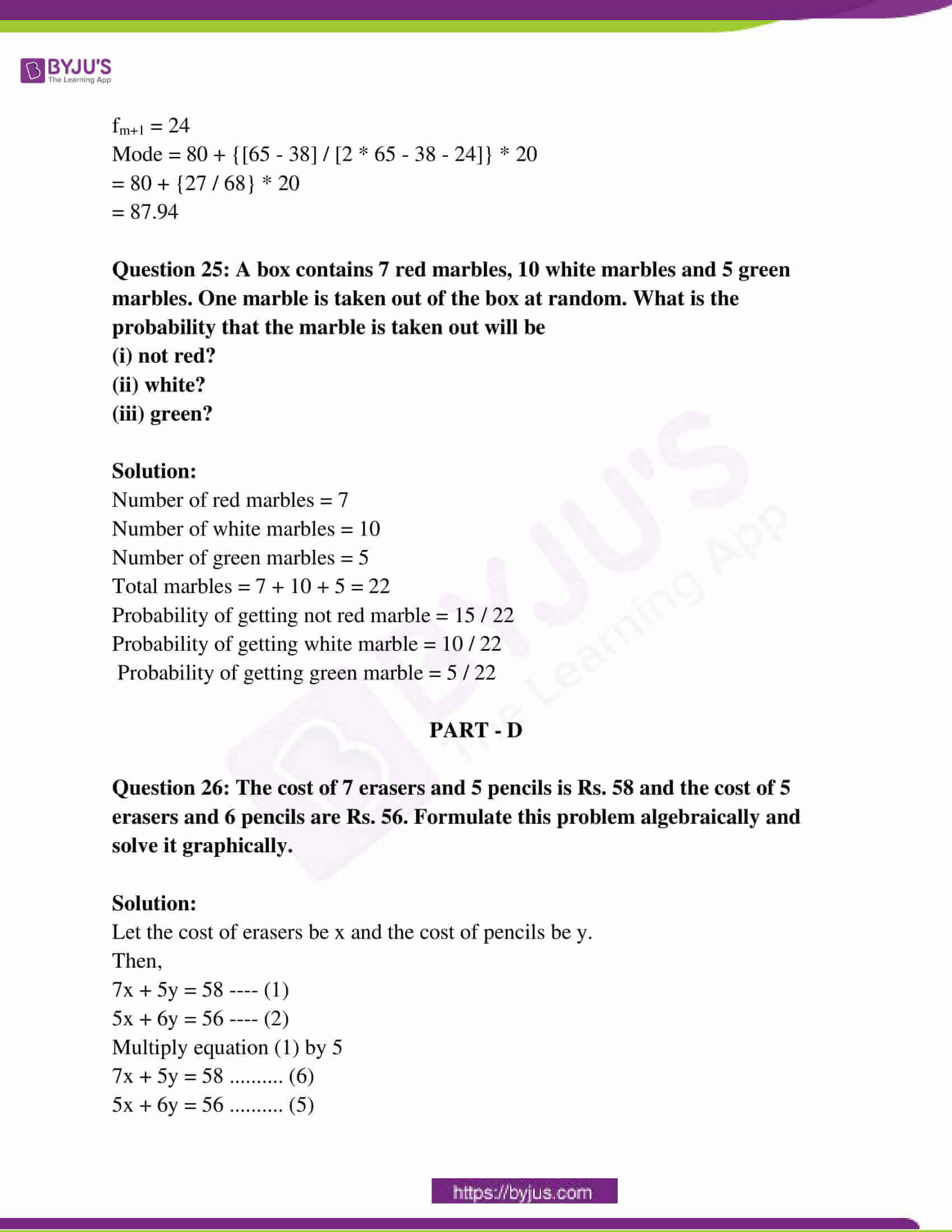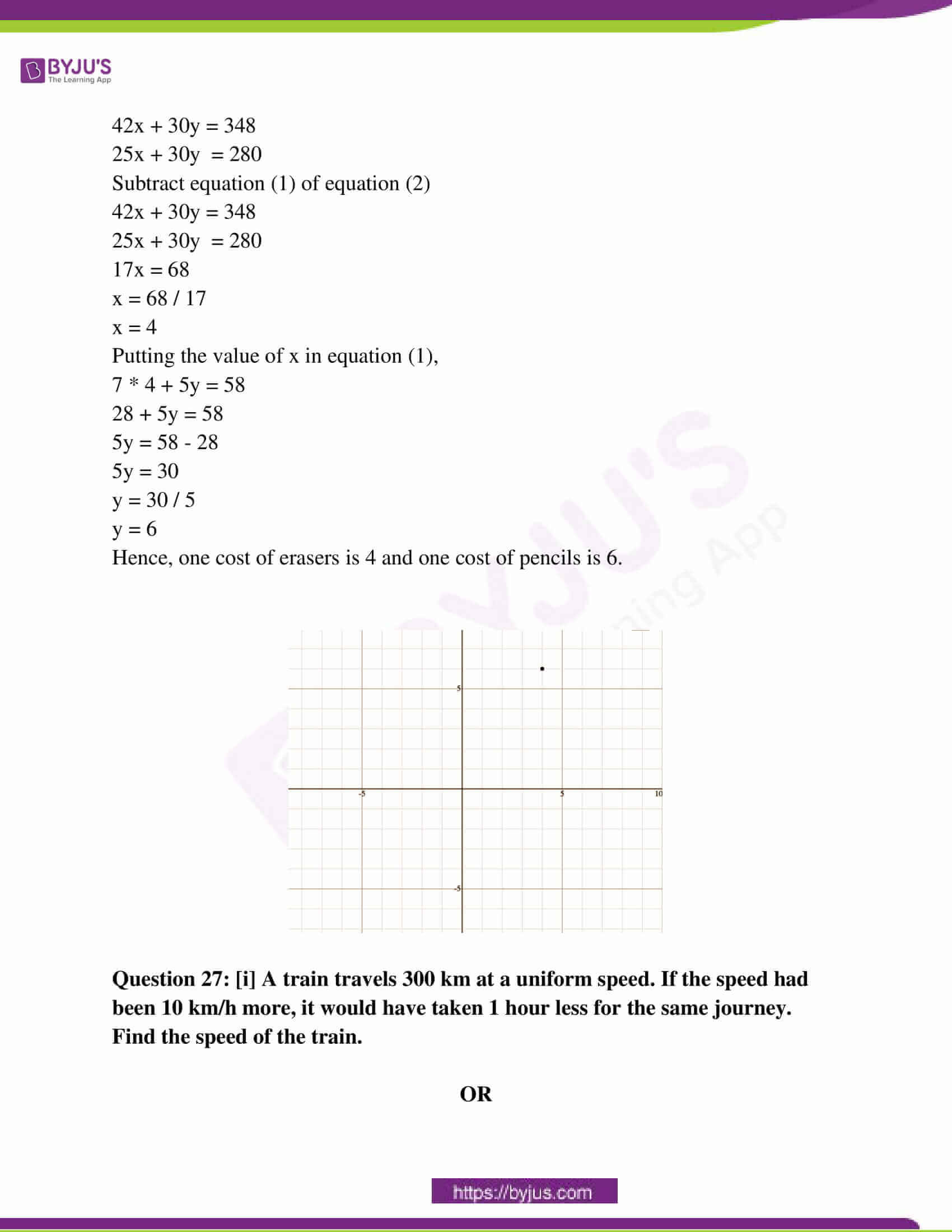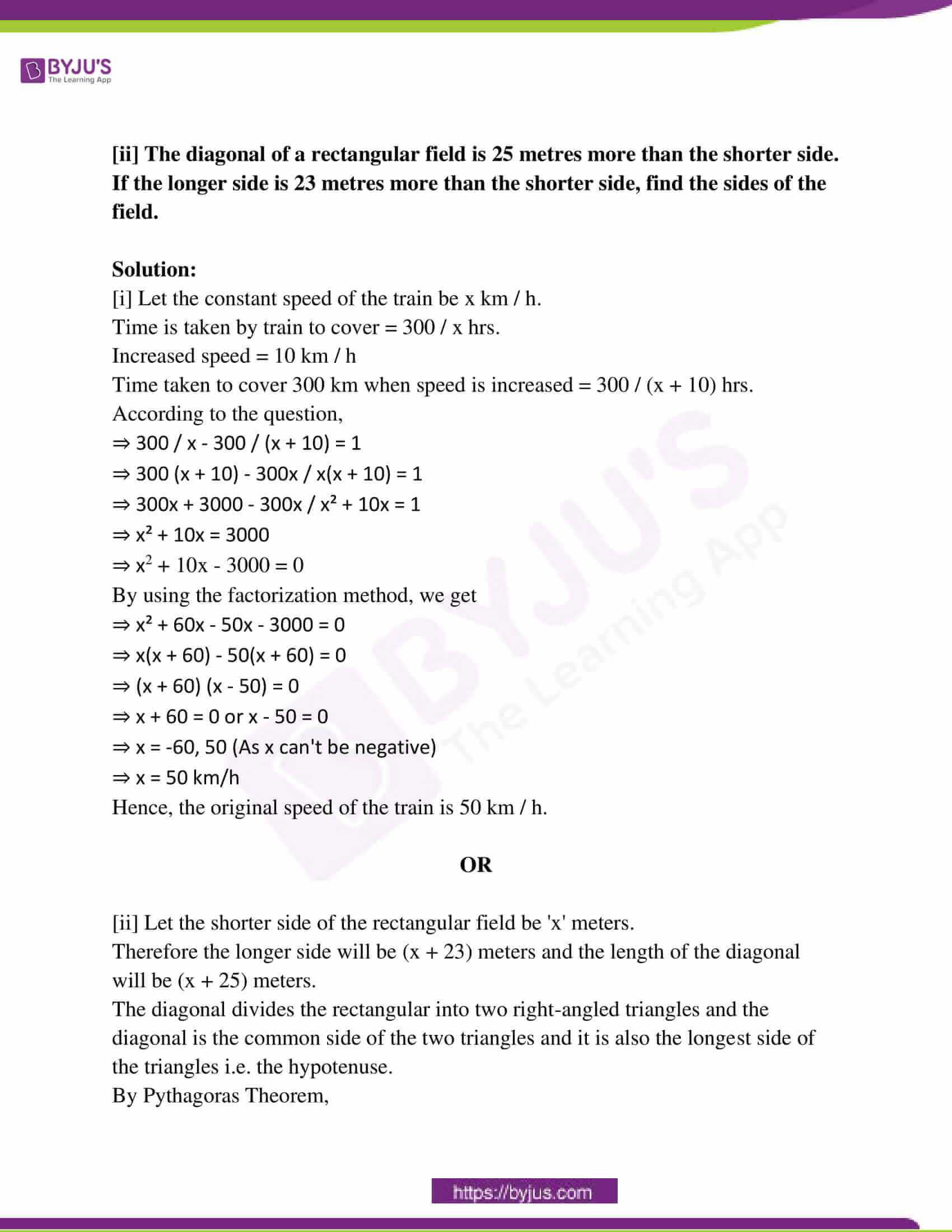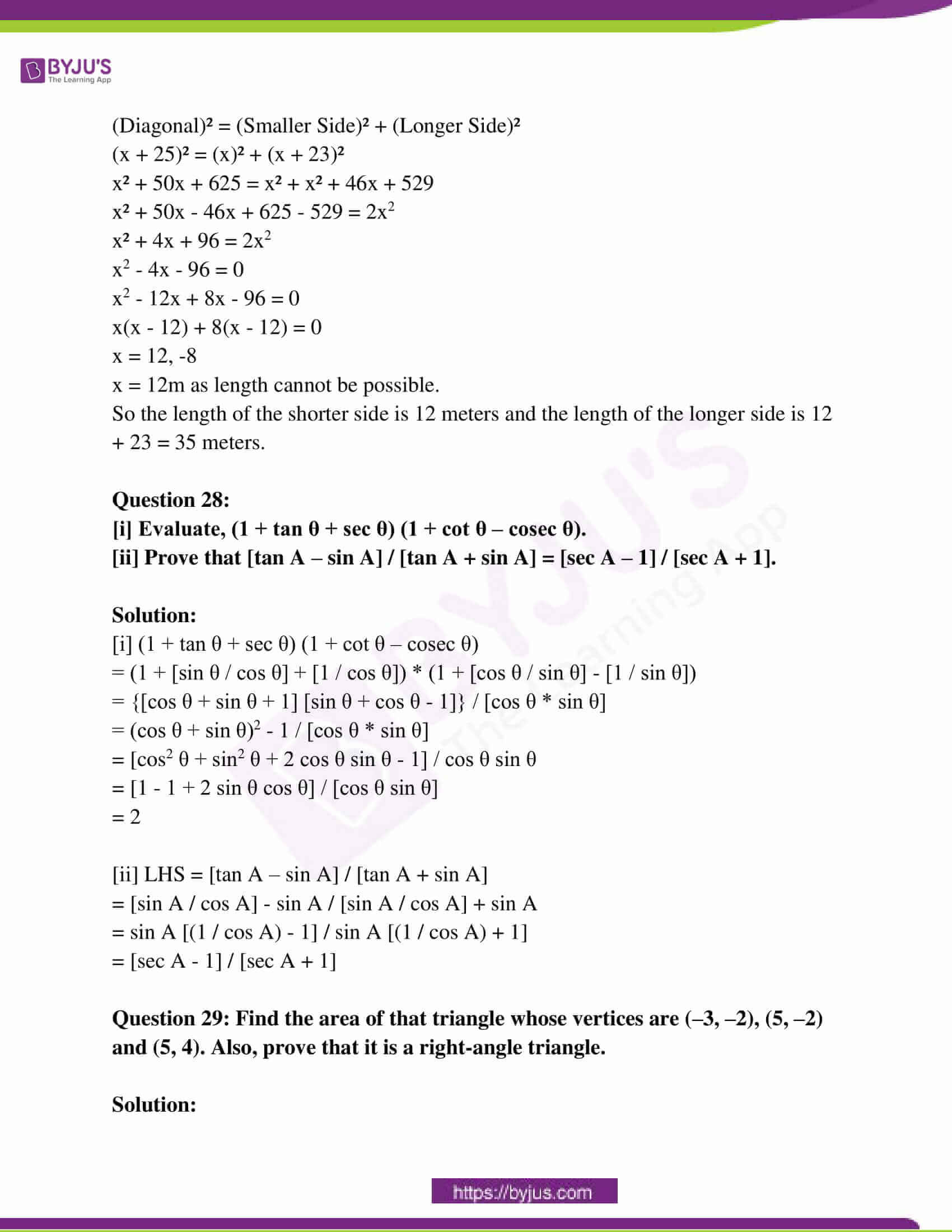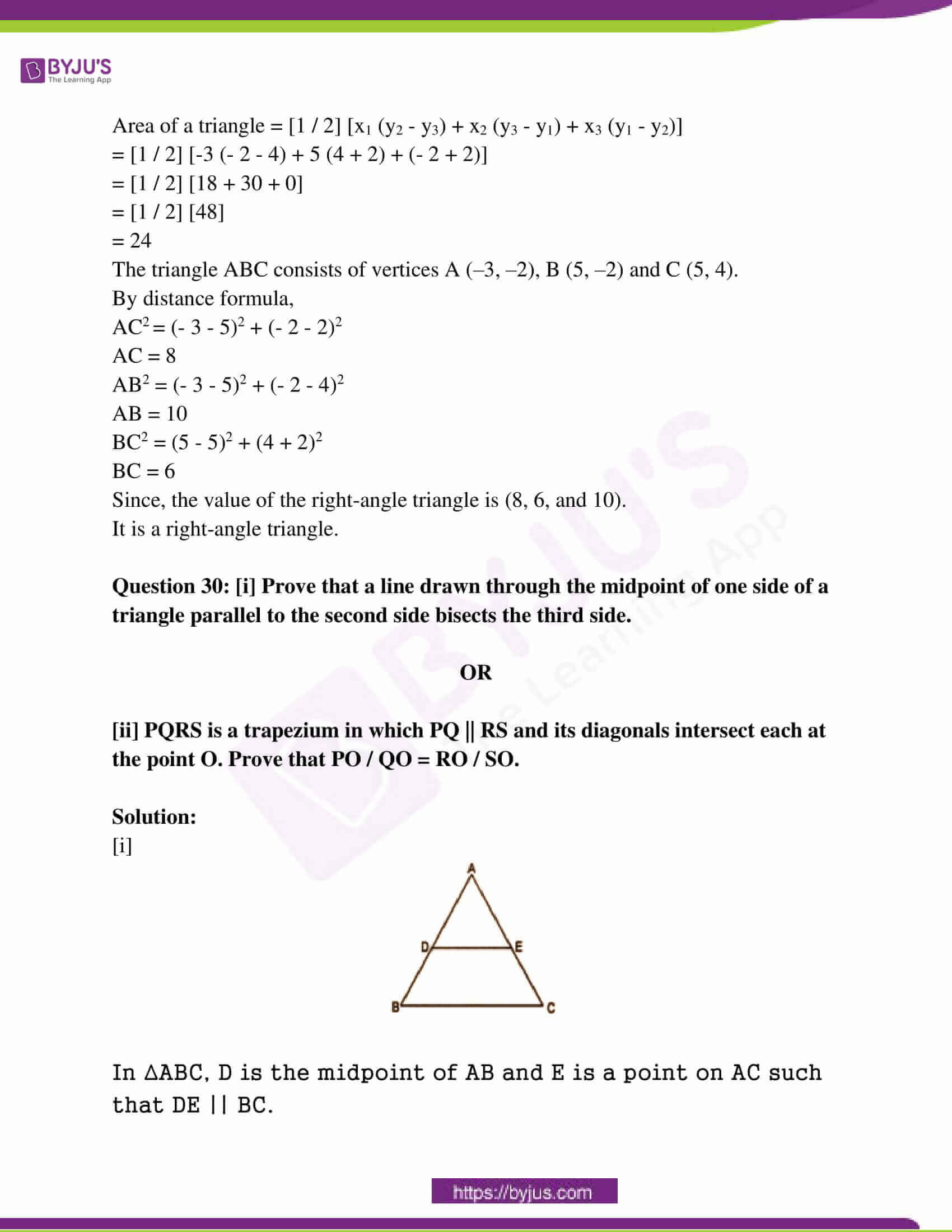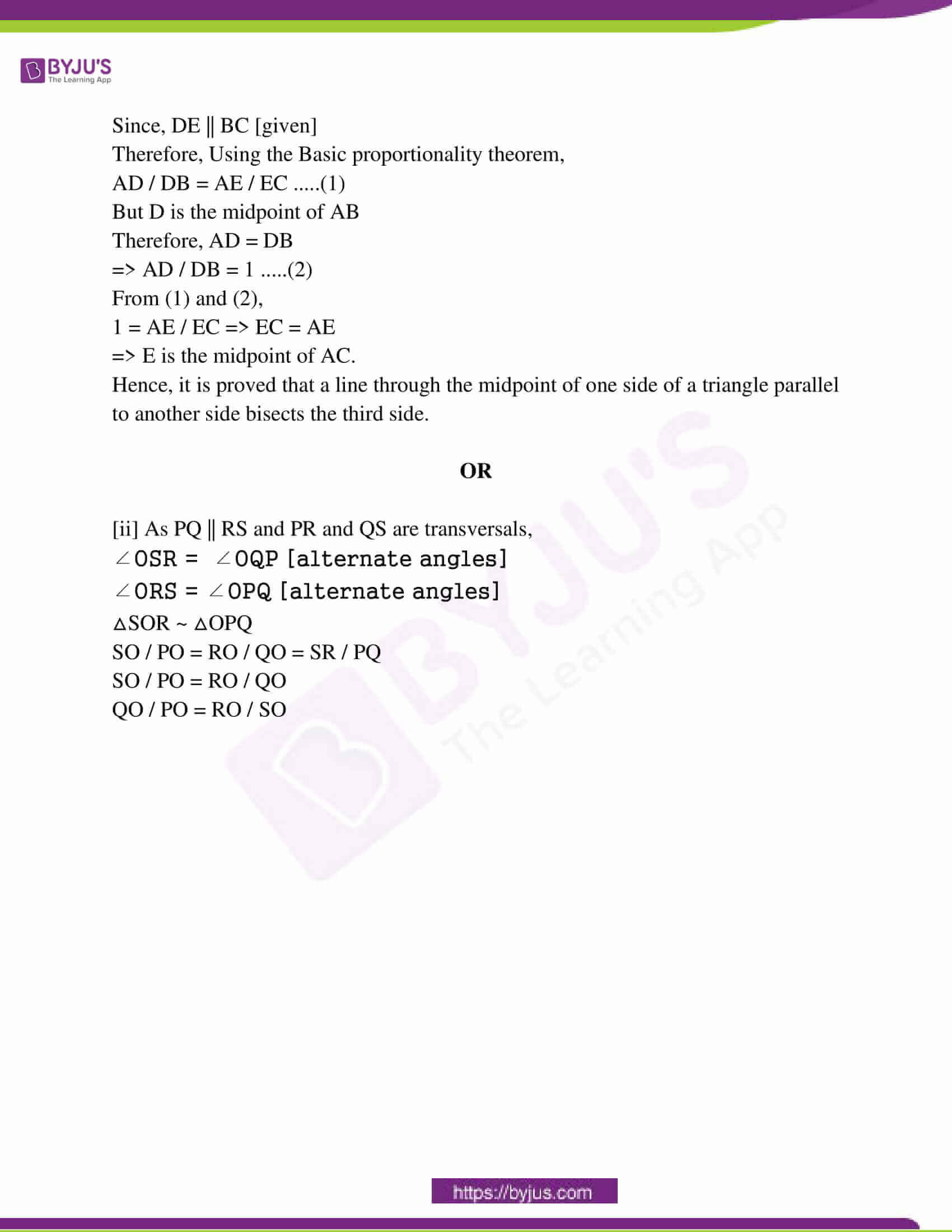### RBSE Class 10th Maths Question Paper With Solution 2017

PART – A

Question 1: HCF and LCM of two integers are 12 and 336, respectively. If one integer is 48, then find another integer.

Solution:

HCF and LCM of two integers are 12 and 336.

First number × Second number = HCF × LCM

Let another integer be a

⇒ 48 × a = 12 × 336

⇒ a = [12 × 336] / 

a = 84

Hence, the second integer is 84.

Question 2: Find the sum of the first 20 terms of the AP: 13, 8, 3, …..

Solution:

a = 13

d = 8 – 13 = -5

n = 20

Sn = [n / 2] [2a + [n – 1] d]

= [20 / 2] (2 * 13 + [20 – 1] * (-5)]

=  [26 – 95]

= 10 * [-69]

= -690

Question 3: If cosec A = 17 / 8, then calculate tan A.

Solution:

cosec A = 17 / 8

h = 17

p = 8

b = ?

p2 + b2 = h2

82 + b2 = 172

b2 = 172 – 82

b2 = 289 – 64

b2 = 225

b = 15

tan A = p / b

= 8 / 15

Question 4: Write the trigonometric ratio of sin A in terms of cot A.

Solution:

sinA= 1 / cosecA

= 1 / √(1 + cot²A)

cot²A + 1 = cosec²A

cosecA= √(1 + cot²A)]

Question 5: If the ratio of corresponding medians of two similar triangles is 9:16, then find the ratio of their areas.

Solution:

The theorem states that “the ratio of the areas of two triangles is equal to the square of the ratio of their corresponding medians”.

Thus, we have,

Ratio of medians = 9:16 = 9 / 16

Ratios of their areas = [9 / 16]2

= 81 / 256

Question 6: From a point Q, the length of the tangent to a circle is 15 cm and the distance of Q from the centre of the circle is 17 cm, then find the radius of the circle.

Solution: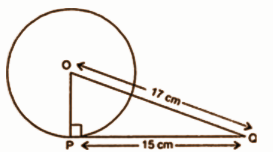PQ = 15cm

OQ = 17cm

OQ2 = OP2 + PQ2

172 = OP2 + 152

172 – 152 = OP2

289 – 225 = OP2

64 = OP2

OP = 8cm

Question 7: Draw a pair of tangents to a circle of radius 5 cm which is inclined to each other at an angle of 70°.

Solution: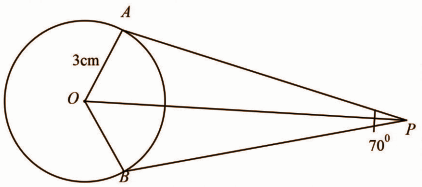Question 8: If the circumference and the area of a circle are numerically equal, then find the radius of the circle.

Solution:

Area of circle = circumference of the circle

=> πr² = 2πr

=> Dividing by πr on both the sides

=> r = 2

=> So the radius of the circle is 2 units.

Question 9: Find the area of a quadrant of a circle whose circumference is 44 cm.

Solution:

Circumference, 2πr = 44

πr = 22

[22 / 7] * r = 22

r = 7 cm

Area of Quadrant = [1 / 4] * πr²

= [1 / 4] * [22 / 7] * 7 * 7

= [22 * 7] / 4

= 154 / 4

= 38.5 cm²

Question 10: If the probability of “not E” = 0.95, then find P(E).

Solution:

P (not E) = 0.95

Thus P (E) = 1 − P (not E)

⇒ P (E) = 1 − 0.95

⇒ P (E) = 0.05

PART – B

Question 11: Name the type of quadrilateral formed by the points (4, 5), (7, 6), (4, 3), (1, 2).

Solution:

Let the points (4, 5), (7, 6), (4, 3), and (1, 2) be representing the vertices A, B, C, and D of the given quadrilateral, respectively.

AB = √(4 – 7)2 + (5 – 6)2 = √(-3)2 + (-1)2 = √9 + 1 = √10

BC = √(7 – 4)2 + (6 – 3)2 = √(3)2 + (3)2 = √9 + 9 = √18

CD = √(4 – 1)2 + (3 – 2)2 = √(3)2 + (1)2 = √9 + 1 = √10

AD = √(4 – 1)2 + (5 – 2)2 = √(3)2 + (3)2 = √9 + 9 = √18

Diagonal AC = √(4 – 4)2 + (5 – 3)2 = √(0)2 + (2)2 = √0 + 4 = 2

Diagonal CD = √(7 – 1)2 + (6 – 2)2 = √(6)2 + (4)2 = √36 + 16 = √52

The opposite sides of this quadrilateral are of the same length. However, the diagonals are of different lengths.

Therefore, the given points are the vertices of a parallelogram.

Question 12: A vessel is in the form of a hollow hemisphere. The diameter of the hemisphere is 14 cm. Find the inner surface area of the vessel.

Solution:

D = 14cm (given)

R = 14 / 2 = 7cm

CSA of hemisphere = 2πr2

= 2 × [22 / 7] × 7 × 7

= 44 × 7

= 308 cm2

Question 13: The following distribution shows the daily pocket allowance of children of a locality.

 Daily pocket allowance 10 – 20 20 – 30 30 – 40 40 – 50 50 – 60 Number of children 3 5 4 7 6

Find the mean daily pocket allowance by using the appropriate method.

Solution:

 Daily pocket allowance 10 – 20 20 – 30 30 – 40 40 – 50 50 – 60 Number of children (fi) 3 5 4 7 6 Midpoint (xi) 15 25 35 45 55 fixi 45 125 140 315 330

Mean = ∑fixi / ∑fi

= 955 / 25

= 38.2

Question 14: A car travels 260 km distance from a place A to place B, at a uniform speed 65 km/hr passes through all thirteen green traffic signals, 4 minutes at the first signal, 7 minutes at the second signal, 10 minutes at a third signal and so on stops for 40 minutes at the thirteenth signal. How much total time does it take to reach place B? Solve by suitable Mathematical Method.

Solution:

Time sequence forms an A.P: 4, 7, 10……

a = 4

d = 7 − 4 = 3

For the 13th station, time = 40

Total time is the sum of the first thirteen terms of the sequence

S = [n / 2] {2a + (n − 1) d}

S = [13 / 2] {2(4) + (13 − 1) 3}

S = [13 / 2] [8 + 36]

S = [13 / 2] × 44

S = 286

S = [286 / 60] hour

= 4 + [286 / 60]

= [240 + 286] / 60

= 526 / 60

= 8.76 hours

Question 15: For traffic control, a CCTV camera is fixed on a straight and vertical pole. The camera can see 113 m distance straight from the top. If the area visible by the camera around the pole is 39424 m2, then find the height of the pole.

Solution:

The height of the pole = 15m

As the area visible by the camera around the pole would form a circle.

Thus, the area visible by the camera around the pole = 𝛑r²

Here, the correct area visible by the camera around the pole = 39424 m²

=> 39424 m² = (22 / 7) * r²

=> r² = 12544

=> r = 112 m

Thus, the radius of circle = 112 m

Also, the camera can see 113 m distance from the top.

So, A right triangle is formed whose base is 112 m and the hypotenuse is 113 m.

Thus, perpendicular = √hypotenuse² – base²

= √113² – 112²

= √225

= 15 m

Thus, the height of the pole = 15 m.

PART – C

Question 16: Prove that √3 is an irrational number.

Solution:

Let us assume that √3 is a rational number.

A rational number should be in the form of p / q, where p and q are a coprime number.

√3 = p / q { where p and q are co- prime}

√3q = p

Now, by squaring both the side we get,

(√3q)² = p²

3q² = p² …….. (i)

So, if 3 is the factor of p² then, 3 is also a factor of p ….. (ii)

=> Let p = 3m { where m is any integer }

Squaring both sides,

p² = (3m)²

p² = 9m²

Putting the value of p² in equation (i)

3q² = p²

3q² = 9m²

q² = 3m²

So, if 3 is a factor of q², then, 3 is also a factor of q.

Since 3 is the factor of p & q both, our assumption that p & q are coprime is wrong.

Hence √3 is an irrational number.

Question 17: Divide x3 – 6x2 + 11x – 6 by x – 2, and verify the division algorithm.

Solution: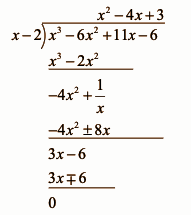Dividend = Quotient * Divisor + Remainder

= (x – 2) (x2 – 4x + 3) + 0

= x3 – 4x2 + 3x – 2x2 + 8x – 6

= x3 – 6x2 + 11x – 6

Question 18: A manufacturer of TV sets produced 720 sets in the fourth year and 1080 sets in the sixth year. Assuming that the production increases uniformly by a fixed number every year, then find total production in the first 9 years.

Solution:

Simplify the expression,

720 set in the fourth year

1080 set in the sixth year

2-year difference value of the sixth year – 4 year = 1080 – 720 = 360

2 difference = 360

1 year produced is = 360 / 2 = 180

Then, 1 year produced is 180

9-year total production are = 180 * 9 = 1620

9 year total production are = 180 * 9 = 1620

9 year total production are = 180 * 9 = 1620

9 year total production are = 1620

Hence, the total production of 9 years is 1620.

Question 19: A tree breaks due to a storm and the broken part bends so that the top of the tree touches the ground making an angle 60° with it. The distance between the foot of the tree to the point where the top touches the ground is 3 m. Find the height of the tree.

Solution: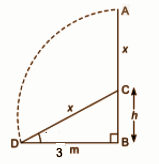Let the height of the tree before the storm is AB.

Due to the storm, it breaks from C such that its top touches the ground at D and makes an angle of 60o.

Let AC = DC = x and BC = h, BD = 3m

Height of the tree = BC = x + h —- (1)

In triangle BCD,

CD / BD = sec 60o and BC / BD = tan 60o

x / 3 = 2 and h / 3 = √3

x = 6 and h = 3√3

Substitute the value of x and h in (1)

BC = 6 + 3√3

= 11.1m

Question 20: Prove that the angle between the two tangents drawn from an external point to a circle is supplementary to the angle subtended by the line-segment joining the points of contact at the centre.

Solution: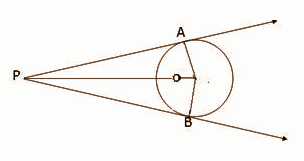Consider a circle with centre O.

Let P be an external point from which two tangents PA and PB are drawn to the circle which are touching the circle at point A and B respectively and AB is the line segment, joining point of contacts A and B together such that it subtends ∠AOB at centre O of the circle.

It can be observed that

OA ⊥ PA

∴ ∠OAP = 90°

Similarly, OB ⊥ PB

∴ ∠OBP = 90°

Sum of all interior angles = 360º

∠OAP + ∠APB + ∠PBO + ∠BOA = 360º

⇒ 90º + ∠APB + 90º + ∠BOA = 360º

⇒ ∠APB + ∠BOA = 180º

∴ The angle between the two tangents drawn from an external point to a circle is supplementary to the angle subtended by the line-segment joining the points of contact at the centre.

Question 21: Draw a circle of radius 5 cm. From a point 13 cm away from its centre, construct the pair of tangents to the circle and measure their length. Also, verify the measurement by actual calculation.

Solution: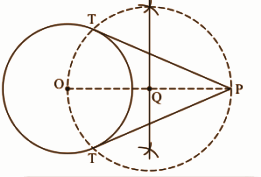Steps of construction:

• Draw a circle of radius OP = 13cm.
• Make a point P at a distance of OP = 13cm.
• Join OP.
• Taking Q as centre and radius OQ = PQ, draw a circle to intersect the given circle at T and T’.
• Join PT and PT’ to obtain the required tangents.
• Thus, PT and P’T’ are the required tangents.

Finding the length of tangents.

We know that OT ⟂ PT and in triangle OPT,

PT2 = OP2 – OT2

= 132 – 52

= 169 – 25

= 144

= 12

The length of the tangents are 12cm each.

Question 22: If an arc of a circle subtends an angle of 60° at the centre and if the area of the minor sector is 231 cm2, then find the radius of the circle.

Solution:

Area of the sector = 231cm2

The angle subtended = 60o

A = 𝛑r2θ / 360o

231 = (22 / 7) * r2 * 60o / 360o

231 = (22 / 7) r2 * 0.1666

231 = 0.5236 r2

r2 = 441

r = 21cm

Question 23: A well of diameter 7 m is dug and earth from digging is evenly spread out to form a platform 22 m × 14 m × 2.5 m. Find the depth of the well.

Solution:

The diameter of the well = 7 m

The radius of the well = 7 / 2 m

L * B * H = πr2h

22 * 14 * 2.5 = 22 / 7 × 7 / 2 × 7 / 2 × h

770 / 38.485 = h

h = 20cm

Question 24: The following data gives information on the observed lifetimes (in hours) of 200 electrical components.

 Lifetime (in hours) 40 – 60 60 – 80 80 – 100 100 – 120 120 – 140 140 – 160 Frequency 25 38 65 24 31 17

Determine the modal lifetimes of the components.

Solution:

As the lifetime (in hours) 80 -100 has a maximum frequency, so it is the modal lifetime in hours.

Mode =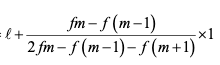Class size h = 20

l = 80

fm = 65

fm-1 = 38

fm+1 = 24

Mode = 80 + {[65 – 38] / [2 * 65 – 38 – 24]} * 20

= 80 + {27 / 68} * 20

= 87.94

Question 25: A box contains 7 red marbles, 10 white marbles and 5 green marbles. One marble is taken out of the box at random. What is the probability that the marble is taken out will be

(i) not red?

(ii) white?

(iii) green?

Solution:

Number of red marbles = 7

Number of white marbles = 10

Number of green marbles = 5

Total marbles = 7 + 10 + 5 = 22

Probability of getting not red marble = 15 / 22

Probability of getting white marble = 10 / 22​

Probability of getting green marble = 5 / 22

PART – D

Question 26: The cost of 7 erasers and 5 pencils is Rs. 58 and the cost of 5 erasers and 6 pencils are Rs. 56. Formulate this problem algebraically and solve it graphically.

Solution:

Let the cost of erasers be x and the cost of pencils be y.

Then,

7x + 5y = 58 —- (1)

5x + 6y = 56 —- (2)

Multiply equation (1) by 5

7x + 5y = 58 ………. (6)

5x + 6y = 56 ………. (5)

42x + 30y = 348

25x + 30y = 280

Subtract equation (1) of equation (2)

42x + 30y = 348

25x + 30y = 280

17x = 68

x = 68 / 17

x = 4

Putting the value of x in equation (1),

7 * 4 + 5y = 58

28 + 5y = 58

5y = 58 – 28

5y = 30

y = 30 / 5

y = 6

Hence, one cost of erasers is 4 and one cost of pencils is 6.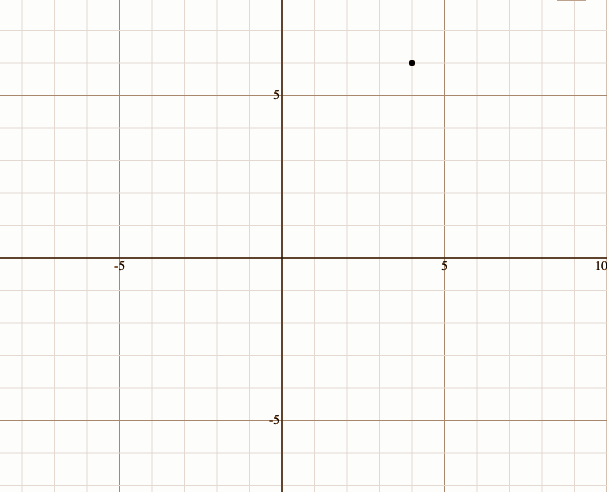Question 27: [i] A train travels 300 km at a uniform speed. If the speed had been 10 km/h more, it would have taken 1 hour less for the same journey. Find the speed of the train.

OR

[ii] The diagonal of a rectangular field is 25 metres more than the shorter side. If the longer side is 23 metres more than the shorter side, find the sides of the field.

Solution:

[i] Let the constant speed of the train be x km / h.

Time is taken by train to cover = 300 / x hrs.

Increased speed = 10 km / h

Time taken to cover 300 km when speed is increased = 300 / (x + 10) hrs.

According to the question,

⇒ 300 / x – 300 / (x + 10) = 1

⇒ 300 (x + 10) – 300x / x(x + 10) = 1

⇒ 300x + 3000 – 300x / x² + 10x = 1

⇒ x² + 10x = 3000

⇒ x2 + 10x – 3000 = 0

By using the factorization method, we get

⇒ x² + 60x – 50x – 3000 = 0

⇒ x(x + 60) – 50(x + 60) = 0

⇒ (x + 60) (x – 50) = 0

⇒ x + 60 = 0 or x – 50 = 0

⇒ x = -60, 50 (As x can’t be negative)

⇒ x = 50 km/h

Hence, the original speed of the train is 50 km / h.

OR

[ii] Let the shorter side of the rectangular field be ‘x’ meters.

Therefore the longer side will be (x + 23) meters and the length of the diagonal will be (x + 25) meters.

The diagonal divides the rectangular into two right-angled triangles and the diagonal is the common side of the two triangles and it is also the longest side of the triangles i.e. the hypotenuse.

By Pythagoras Theorem,

(Diagonal)² = (Smaller Side)² + (Longer Side)²

(x + 25)² = (x)² + (x + 23)²

x² + 50x + 625 = x² + x² + 46x + 529

x² + 50x – 46x + 625 – 529 = 2x2

x² + 4x + 96 = 2x2

x2 – 4x – 96 = 0

x2 – 12x + 8x – 96 = 0

x(x – 12) + 8(x – 12) = 0

x = 12, -8

x = 12m as length cannot be possible.

So the length of the shorter side is 12 meters and the length of the longer side is 12 + 23 = 35 meters.

Question 28:

[i] Evaluate, (1 + tan θ + sec θ) (1 + cot θ – cosec θ).

[ii] Prove that [tan A – sin A] / [tan A + sin A] = [sec A – 1] / [sec A + 1].

Solution:

[i] (1 + tan θ + sec θ) (1 + cot θ – cosec θ)

= (1 + [sin θ / cos θ] + [1 / cos θ]) * (1 + [cos θ / sin θ] – [1 / sin θ])

= {[cos θ + sin θ + 1] [sin θ + cos θ – 1]} / [cos θ * sin θ]

= (cos θ + sin θ)2 – 1 / [cos θ * sin θ]

= [cos2 θ + sin2 θ + 2 cos θ sin θ – 1] / cos θ sin θ

= [1 – 1 + 2 sin θ cos θ] / [cos θ sin θ]

= 2

[ii] LHS = [tan A – sin A] / [tan A + sin A]

= [sin A / cos A] – sin A / [sin A / cos A] + sin A

= sin A [(1 / cos A) – 1] / sin A [(1 / cos A) + 1]

= [sec A – 1] / [sec A + 1]

Question 29: Find the area of that triangle whose vertices are (–3, –2), (5, –2) and (5, 4). Also, prove that it is a right-angle triangle.

Solution:

Area of a triangle = [1 / 2] [x1 (y2 – y3) + x2 (y3 – y1) + x3 (y1 – y2)]

= [1 / 2] [-3 (- 2 – 4) + 5 (4 + 2) + (- 2 + 2)]

= [1 / 2] [18 + 30 + 0]

= [1 / 2] 

= 24

The triangle ABC consists of vertices A (–3, –2), B (5, –2) and C (5, 4).

By distance formula,

AC2 = (- 3 – 5)2 + (- 2 – 2)2

AC = 8

AB2 = (- 3 – 5)2 + (- 2 – 4)2

AB = 10

BC2 = (5 – 5)2 + (4 + 2)2

BC = 6

Since, the value of the right-angle triangle is (8, 6, and 10).

It is a right-angle triangle.

Question 30: [i] Prove that a line drawn through the midpoint of one side of a triangle parallel to the second side bisects the third side.

OR

[ii] PQRS is a trapezium in which PQ || RS and its diagonals intersect each at the point O. Prove that PO / QO = RO / SO.

Solution:

[i]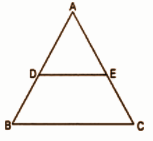In ∆ABC, D is the midpoint of AB and E is a point on AC such that DE || BC.

DE || BC [given]

Therefore, Using the Basic proportionality theorem,

AD / DB = AE / EC …..(1)

But D is the midpoint of AB

=> AD / DB = 1 …..(2)

From (1) and (2),

1 = AE / EC => EC = AE

=> E is the midpoint of AC.

Hence, it is proved that a line through the midpoint of one side of a triangle parallel to another side bisects the third side.

OR

[ii] As PQ || RS and PR and QS are transversals,

∠OSR = ∠OQP [alternate angles]

∠ORS = ∠OPQ [alternate angles]

△SOR ~ △OPQ

SO / PO = RO / QO = SR / PQ

SO / PO = RO / QO

QO / PO = RO / SO